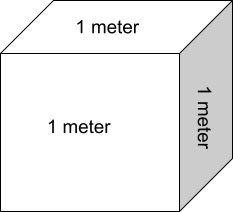# Cubic Meter Calculator

To find cubic meter, input length, width, & height and press calculate button using cubic meter calculatorInput the values of length, width, and height in the cubic meters (cbm) calculator to find the volume of a cube.

The cubic meter calculator finds the volume of a cube in cbm and also converts it into centimeters (cm), kilograms (kg), yards, and Cubic feet (cft).

Along with converting cbm to multiple units, this tool gives a labeled diagram with volume in meters written in the center.

## What is CBM?

CBM (meaning cubic meter) is the SI unit of volume and its symbol is “m3”.

Cubic meter refers to the volume of a cube with all three sides (length, width, and height) of one meter.## How to calculate CBM?

To compute the cubic capacity, you need to measure different dimensions i.e length, width, and height of the object. After measuring, put them in the formula.

Formula:

• Length x Width x Height
• Cm to cbm: (L x W x H) 100000
• Cft to cbm: (L x W x H) 35.31

Example:

Calculate:Solution:

Step 1: Identify the values.

Length = 13m

Width = 13m

Height = 20m

Step 2: Multiply.

= 13 x 13 x 20

= 3380 m3